Students can download Maths Chapter 8 Statistics Additional Questions and Answers, Notes, Samacheer Kalvi 9th Maths Guide Pdf helps you to revise the complete Tamilnadu State Board New Syllabus, helps students complete homework assignments and to score high marks in board exams.

## Tamilnadu Samacheer Kalvi 9th Maths Solutions Chapter 8 Statistics Additional Questions

Question 1.
The Arithmetic mean of all the factors of 10 is ………
(a) 4.5
(b) 5.5
(c) 10
(d) 55
Solution:
(a) 4.5

Question 2.
The mean of five numbers is 27, if one number is excluded, then mean is 25. Then the excluded number is ……..
(a) 0
(b) 15
(c) 25
(d) 35
Solution:
(d) 35Question 3.
The mean of 8 numbers is 15. If each number is multiplied by 2, then the new mean will be ……..
(a) 7.5
(b) 30
(c) 10
(d) 25
Solution:
(b) 30

Question 4.
The median of 11, 8, 4, 9, 7, 5, 2, 4, 10 is ……..
(a) 1
(b) 8
(c) 4
(d) 11
Solution:
(a) 1Question 5.
Median is …….
(a) the most frequent value
(b) the least frequent value
(c) middle most value
(d) mean of first and last values
Solution:
(c) middle most value

Question 6.
The mode of the distribution is …….(a) 3
(b) 4
(c) 6
(d) 14
Solution:
(b) 4Question 7.
Mode is …….
(a) the middle value
(b) extreme value
(c) minimum value
(d) the most repeated value
Solution:
(d) the most repeated value

Question 8.
The mode of the data 72, 33, 44, 72, 81, 72, 15 is ……..
(a) 12
(b) 33
(c) 81
(d) 15
Solution:
(a) 12Question 9.
The Arithmetic mean of 10 number is -7. If 5 is added to every number, then the new arithmetic mean is ……..
(a) 17
(b) 12
(c) -2
(d) -7
Solution:
(c) -2

Question 10.
The Arithmetic mean of integers from -5 to 5 is ……..
(a) 25
(b) 10
(c) 3
(d) 0
Solution:
(d) 0Question 1.
Find the Arithmetic mean for the following data.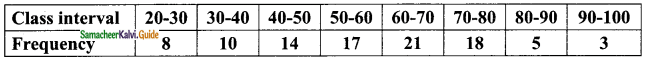Solution:Arithmetic mean ($$\bar { X }$$) = $$\frac{Σfx}{Σf}$$
= $$\frac{5540}{96}$$
= 57.7
∴ Arithmetic mean = 57.7

Question 2.
Calculate the Arithmetic mean of the following data using step deviation method.Solution:
Assumed mean = 35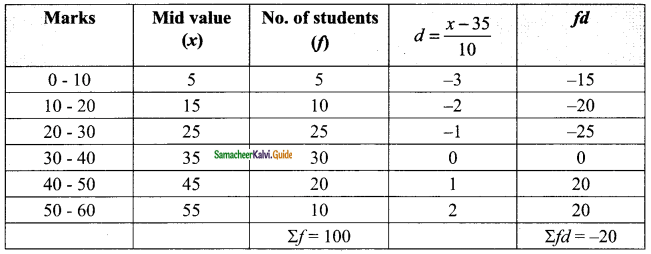Arithmetic mean ($$\bar { X }$$) = A + $$\frac{Σfd}{Σf}$$ × c
= 35 + $$\frac{(-20)}{100}$$ × 10
= 35 – 2
= 33
∴ Arithmetic mean = 33Question 3.
Find the median for the following data.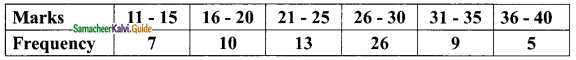Solution:
The given class intervals is inclusive type we convert it into exclusive type.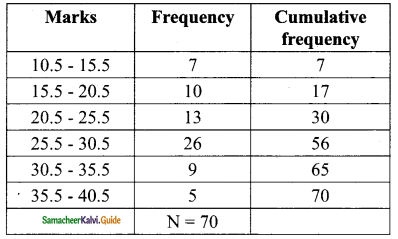$$\frac{N}{2}$$ = $$\frac{70}{2}$$
= 35
Median class is 25.5 – 30.5
Here l = 25.5, f = 26, m = 30, c = 5
Median = l + $$\frac{\frac{N}{2}-m}{f}$$ × c
= 25.5 + $$\frac{35-30}{26}$$ × 5
= 25.5 + $$\frac{5×5}{26}$$
= 25.5 + 0.96
= 26.46
∴ Median = 26.46Question 4.
Calculate the mode of the following data.Solution: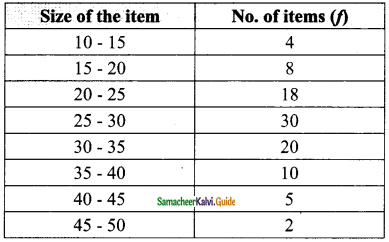The highest frequency is 30. Corresponding class interval is the modal class.
Here l = 25, f = 30, f1 = 18, f2 = 20 and c = 5
Mode∴ Arithmetic mean = 25 + 2.727
= 25 + 2.73
= 27.73
mode = 27.73Question 5.
Find the mean, median and mode of marks obtained by 20 students in an examination. The marks are given below.Solution: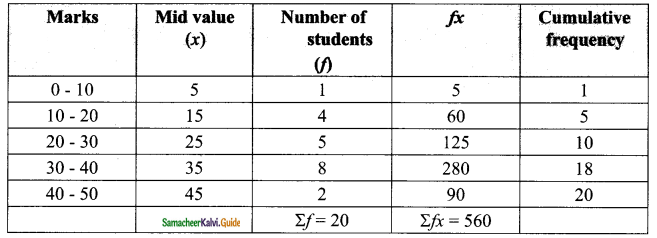Arithmetic mean:
Here Σfx = 560, Σf = 20
$$\bar { X }$$ = $$\frac{Σfx}{Σf}$$
= $$\frac{560}{20}$$

Median:
Median class is 20 – 30
Here l = 20, $$\frac{N}{2}$$ = 10, m = 5, c = 10, f = 5
Median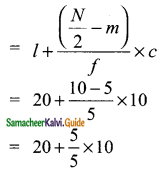= 20 + 10
= 30

Mode:
The highest frequency is 8
Modal class is 30 – 40
Here, l = 30, f = 8, f1 = 5, f2 = 2, c = 10
Mode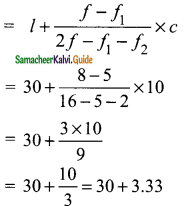= 33.33
Mean = 28, median = 30, mode = 33.33